Documentation

# Eye Diagram Scope

Display eye diagram of time-domain signal

## Library

Comm Sinks## Description

The Eye Diagram block displays multiple traces of a modulated signal to produce an eye diagram. You can use the block to reveal the modulation characteristics of the signal, such as the effects of pulse shaping or channel distortions.

The Eye Diagram block has one input port. This block accepts a column vector or scalar input signal. The block accepts a signal with the following data types: double, single, base integer, and fixed point. All data types are cast as double before the block displays results.## Dialog Box

To modify the eye diagram display, select View > Configuration Properties or click the button (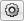). Then select the Main, 2D color histogram, Axes, or Export tabs and modify the settings.

## Visuals — Eye Diagram Properties

### Main Tab#### Display mode

Display mode of the eye diagram, specified as `Line plot` or `2D color histogram`. Selecting ```2D color histogram``` makes the histogram tab available. This parameter is tunable.

#### Enable measurements

Select this check box to enable eye measurements of the input signal.

#### Show horizontal (jitter) histogram

Select this radio button to display the jitter histogram. This parameter is available when Display mode is `2D color histogram` and Enable measurements is selected. This can also be accessed by using the histogram button drop down on the toolbar.

#### Show vertical (noise) histogram

Select this radio button to display the noise histogram. This parameter is available when Display mode is `2D color histogram` and Enable measurements is selected. This can also be accessed by using the histogram button drop down on the toolbar.

#### Show horizontal bathtub curve

Select this check box to display the horizontal bathtub curve. This parameter is available when Enable measurements is selected. This can also be accessed by using the bathtub curve button on the toolbar.

#### Show vertical bathtub curve

Select this check box to display the vertical bathtub curve. This parameter is available when Enable measurements is selected. This can also be accessed by using the bathtub curve button on the toolbar.

#### Eye diagram to display

Select either `Real only` or ```Real and imaginary``` to display one or both eye diagrams. To make eye measurements, this parameter must be `Real only`. This parameter is tunable.

Select this check box to fade the points in the display as the interval of time after they are first plotted increases. The default value is `false`. This parameter is available only when the Display mode is `Line plot`. This property is tunable.

#### Samples per symbol

Number of samples per symbol. Use with Symbols per trace to determine the number of samples per trace. This parameter is tunable.

#### Sample offset

Sample offset, specified as a nonnegative integer smaller than the product of Samples per symbol and Symbols per trace. The offset provides the number of samples to omit before plotting the first point. This parameter is tunable.

#### Symbols per trace

Number of symbols plotted per trace, specified as a positive integer. This parameter is tunable.

#### Traces to display

Number of traces plotted. This parameter is available only when the Display mode is `Line plot`. This parameter is tunable.

### Axes Tab#### Title

Label that appears above the eye diagram plot. By default, the plot has no title. This parameter is tunable.

#### Show grid

Toggle this check box to turn the grid on and off. This parameter is tunable.

#### Y-limits (Minimum)

Minimum value of the y-axis. This parameter is tunable.

#### Y-limits (Maximum)

Maximum value of the y-axis. This parameter is tunable.

#### Real axis label

Text that the scope displays along the real axis. This parameter is tunable.

#### Imaginary axis label

Text that the scope displays along the imaginary axis. This parameter is tunable.

### 2D Histogram Tab

The 2D histogram tab is available when you click the histogram button or when the display mode is set to `2D color histogram`.#### Oversampling method

Oversampling method, specified as `None`, `Input interpolation`, or ```Histogram interpolation```. This parameter is tunable.

To plot eye diagrams as quickly as possible, set the Oversampling method to `None`. The drawback to not oversampling is that the plots look pixelated when the number of samples per trace is small. To create smoother, less-pixelated plots using a small number of samples per trace, set the Oversampling method to `Input interpolation` or ```Histogram interpolation```. `Input interpolation` is the faster of the two interpolation methods and produces good results when the signal-to-noise ratio (SNR) is high. With a lower SNR, this oversampling method is not recommended because it introduces a bias to the centers of the histogram ranges. `Histogram interpolation` is not as fast as the other techniques, but it provides good results even when the SNR is low.

#### Color scale

Color scale of the histogram plot, specified as either `Linear` or `Logarithmic`. Set Color scale to `Logarithmic` if certain areas of the eye diagram include a disproportionate number of points. This parameter is tunable.

#### Reset

The toolbar contains a histogram reset button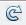, which resets the internal histogram buffers and clears the display. This button is not available when the display mode is set to `Line plot`.

### Export Tab#### Export measurements

Select this check box export the eye diagram measurements to the MATLAB® workspace. This parameter is tunable.

#### Variable name

Specify the name of the variable to which the eye diagram measurements are saved. The default is `EyeData`. This parameter is tunable. The data is saved as a structure having these fields:

• `MeasurementSettings`

• `Measurements`

• `JitterHistogram`

• `NoiseHistogram`

• `HorizontalBathtub`

• `VerticalBathtub`

• `BlockName`

## Style Dialog Box

In the Style dialog box, you can customize the style of the active display. You can change the color of the figure containing the displays, the background and foreground colors of display axes, and properties of lines in a display. To open this dialog box, select View > Style.### Properties

#### Figure color

Specify the background color of the scope figure. By default, the figure color is black.

#### Axes colors

Specify the fill and line colors for the axes.

#### Line

Specify the line style, line width, and line color for the displayed signal.

#### Marker

Specify data point markers for the selected signal. This parameter is similar to the `Marker` property for MATLAB Handle Graphics® plot objects.

SpecifierMarker Type
noneNo marker (default)CircleSquareCrossPointPlus signAsteriskDiamond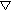Downward-pointing triangleUpward-pointing triangle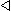Left-pointing triangleRight-pointing triangleFive-pointed star (pentagram)Six-pointed star (hexagram)

#### Colormap

Specify the colormap of the histogram plots as one of these schemes: `Parula`, `Jet`, `HSV`, `Hot`, `Cool`, `Spring`, `Summer`, `Autumn`, `Winter`, `Gray`, `Bone`, `Copper`, `Pink`, `Lines`, or `Custom`. This parameter is active when the Eye Diagram is in `Histogram` mode. The default is `Hot`. If you select `Custom`, a dialog box pops up from which you can enter code to specify your own colormap.## Measurements

expand all

To open the measurements panel, click on the button or select Tools > Measurements > Eye Measurements from the toolbar menu.

### Note

• For amplitude measurements, at least one bin per vertical histogram must reach 10 hits before the measurement is taken, ensuring higher accuracy.

• For time measurements, at least one bin per horizontal histogram must reach 10 hits before the measurement is taken.

• When an eye crossing time measurement falls within the [-0.5/Fs, 0) seconds interval, the time measurement wraps to the end of the eye diagram, i.e., the measurement wraps by 2*Ts seconds (where Ts is the symbol time). For a complex signal case, the analyze method issues a warning if the crossing time measurement of the in-phase branch wraps while that of the quadrature branch does not (or vice versa). To avoid the time-wrapping or a warning, add a half-symbol duration delay to the current value in the `MeasurementDelay` property of the eye diagram object. This additional delay repositions the eye in the approximate center of the scope.

Eye level is the amplitude level used to represent data bits. For the displayed NRZ signal, the levels are –1 V and +1 V. The eye levels are calculated by averaging the 2-D histogram within the eye level boundaries.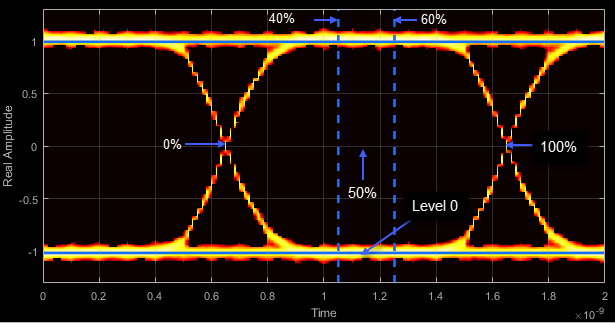Eye amplitude is the distance in V between the mean value of two eye levels.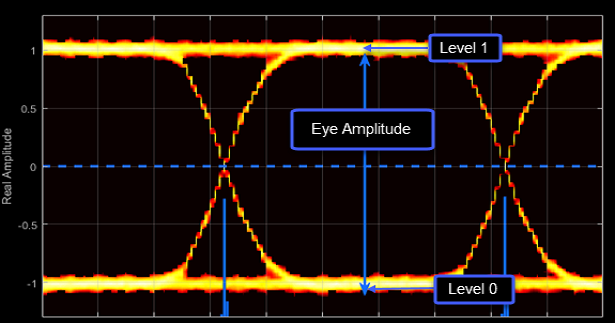Eye height is the distance between μ – 3σ of the upper eye level and μ + 3σ of the lower eye level. μ is the mean of the eye level and σ is the standard deviation.The vertical opening is the distance between the two points that correspond to the BER threshold. For example, for a BER threshold of 10–12, these points correspond to the 7σ distance from each eye level.The eye SNR is the ratio of the eye level difference to the difference of the vertical standard deviations corresponding to each eye level:

`$\text{SNR}=\frac{{L}_{1}-{L}_{0}}{{\sigma }_{1}-{\sigma }_{0}}\text{\hspace{0.17em}},$`

where L1 and L0 represent the means of the upper and lower eye levels and σ1 and σ0 represent their standard deviations.

The Q factor is calculated using the same formula as the Eye SNR. However, the standard deviations of the vertical histograms are replaced with those computed with the dual-Dirac analysis.

The crossing levels are the amplitude levels at which the eye crossings occur.The crossing times are the times at which the crossings occur. The times are computed as the mean values of the horizontal (jitter) histograms.Eye delay is the midpoint between the two crossing times.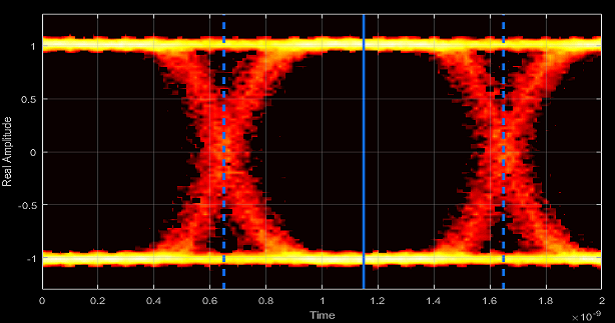Eye width is the horizontal distance between μ + 3σ of the left crossing time and μ – 3σ of the right crossing time. μ is the mean of the jitter histogram and σ is the standard deviation.The horizontal opening is the distance between the two points that correspond to the BER threshold. For example, for a 10–12 BER, these two points correspond to the 7σ distance from each crossing time.Rise time is the mean time between the low and high thresholds defined in the eye diagram. The default thresholds are 10% and 90% of the eye amplitude.Fall time is the mean time between the high and low thresholds defined in the eye diagram. The default thresholds are 10% and 90% of the eye amplitude.The deterministic jitter (DJ) is the distance between the two peaks of the dual-Dirac histograms. The probability density function (PDF) of DJ is composed of two delta functions.The random jitter (RJ) is the Gaussian unbounded jitter component. The random component of jitter is modeled as a zero-mean Gaussian random variable with a specified standard-deviation, σ. The random jitter is computed as:

`$\text{RJ}=\left({Q}_{L}+{Q}_{R}\right)\sigma \text{\hspace{0.17em}},$`

where

`$Q=\sqrt{2}{\mathrm{erfc}}^{-1}\left(2\frac{BER}{\rho }\right)\text{\hspace{0.17em}}.$`

BER is the specified BER threshold. ρ is the amplitude of the left and right Dirac function, which is determined from the bin counts of the jitter histograms.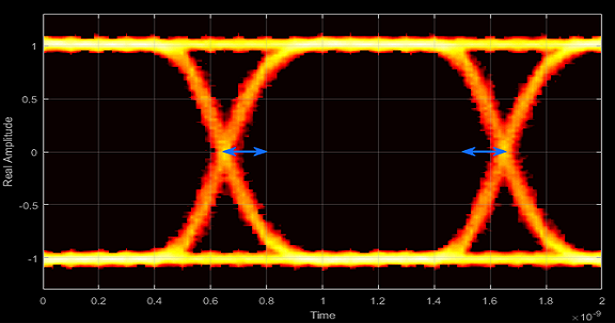Total jitter (TJ) is the sum of the deterministic and random jitter, such that TJ = DJ + RJ.The total jitter PDF is the convolution of the DJ PDF and the RJ PDF.RMS jitter is the standard deviation of the jitter calculated in the horizontal (jitter) histogram at the decision boundary.Peak-to-peak jitter is the maximum horizontal distance between the left and right nonzero values in the horizontal histogram of each crossing time.## Measurement Settings

expand all

To change measurement settings, first select Enable measurements. Then, in the Eye Measurements pane, click the arrow next to Settings. You can control these measurement settings.

`[40 60]` (default) | two-element vector

Time range for calculating eye levels, specified as a two-element vector. These values are expressed as a percentage of the symbol duration. Tunable.

`0` (default) | scalar

Amplitude level threshold in V, specified as a scalar. This parameter separates the different signaling regions for horizontal (jitter) histograms. This parameter is tunable, but the jitter histograms reset when the parameter changes.

For non-return-to-zero (NRZ) signals, set Decision boundary to 0. For return-to-zero (RZ) signals, set Decision boundary to half the maximum amplitude.

`[10 90]` (default) | two-element vector

Amplitude levels of the rise and fall transitions, specified as a two-element vector. These values are expressed as a percentage of the eye amplitude. This parameter is tunable, but the crossing histograms of the rise and fall thresholds reset when the parameter changes.

`0` (default) | scalar

Amplitude tolerance of the horizontal crossings in V, specified as a scalar. Increase hysteresis to provide more tolerance to spurious crossings due to noise. This parameter is tunable, but the jitter and the rise and fall histograms reset when the parameter changes.

`1e-12` (default) | nonnegative scalar from 0 to 0.5

BER used for eye measurements, specified as a nonnegative scalar from 0 to 0.5. The value is used to make measurements of random jitter, total jitter, horizontal eye openings, and vertical eye openings. Tunable.

```[0.5 0.1 0.01 0.001 0.0001 1e-05 1e-06 1e-07 1e-08 1e-09 1e-10 1e-11 1e-12]``` (default) | vector

BER values used to calculate openings of bathtub curves, specified as a vector whose elements range from 0 to 0.5. Horizontal and vertical eye openings are calculated for each of the values specified by this parameter. To enable this parameter, select Show horizontal bathtub curve, Show vertical bathtub curve, or both. Tunable.

`0` (default) | nonnegative scalar

Duration of initial data discarded from measurements, in seconds, specified as a nonnegative scalar.

## Examples

expand all

Display the eye diagram of a filtered QPSK signal using the Eye Diagram block.

Load the doc_eye_diagram_scope model from the MATLAB command prompt.

`doc_eye_diagram_scope`Run the model and observe that two symbols are displayed.Open the configuration parameters dialog box. Change the Symbols per trace parameter to 4. Run the simulation and observe that four symbols are displayed.Try changing the Raised Cosine Transmit Filter parameters or changing additional Eye Diagram parameters to see their effects on the eye diagram.

Display histogram plots of a noisy GMSK signal.

Load the doc_eye_diagram_gmsk model from the MATLAB command prompt.

`doc_eye_diagram_gmsk`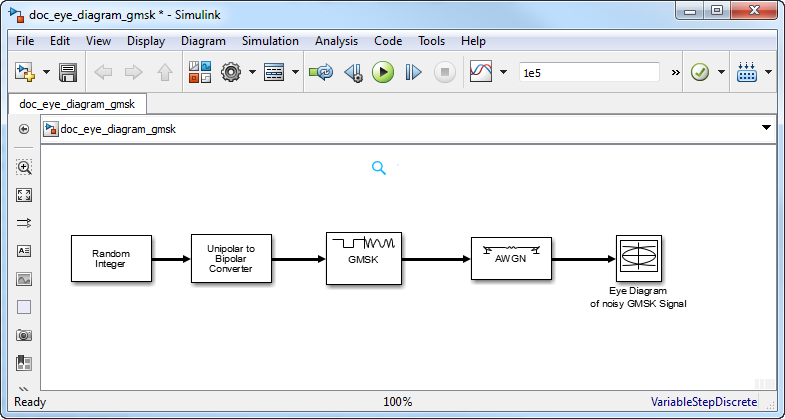Run the model. The eye diagram is configured to show a histogram without interpolation.The lack of interpolation results in a plot having piecewise-continuous behavior.

Open the 2D Histogram tab of the Configuration Properties dialog box. Set the Oversampling method to `Input interpolation`. Run the model.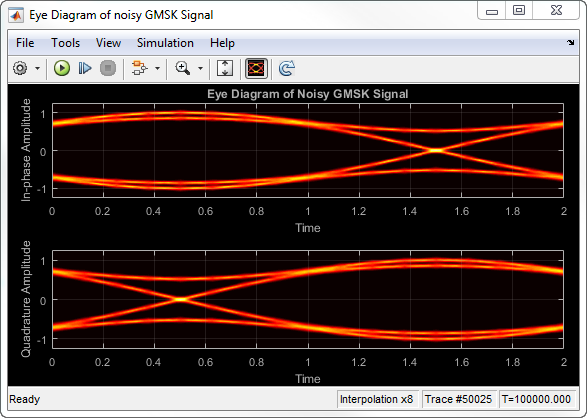The interpolation smooths the eye diagram.

On the AWGN Channel block, change SNR (dB) from `25` to `10`. Run the model.Observe that vertical striping is present in the eye diagram. This striping is the result of input interpolation, which has limited accuracy in low-SNR conditions.

Set the Oversampling method to ```Histogram interpolation```. Run the model.The eye diagram plot now renders accurately because the histogram interpolation method works for all SNR values. This method is not as fast as the other techniques and results in increased execution time.

The `doc_visualize_jitter` model generates bipolar data, adds deterministic and random jitter, applies white noise, and displays the resulting eye diagram.In the `Channel Model with Jitter` block, set the Deterministic jitter parameter to `0` and set the RMS jitter parameter to `0`. When the model runs, the signal shows clean crossings as there is no jitter.Set the Deterministic jitter parameter to `100e-12`. Run the model to show the effect of the deterministic jitter. The separation between the two peaks in the jitter histogram indicates the deterministic jitter.Set the Deterministic jitter parameter to `0` and set the RMS jitter parameter to `50e-12`. Run the model to show the effect of the RMS jitter. The fuzziness around each of the crossings indicates the RMS jitter.Set the Deterministic jitter parameter to `50e-12` and set the RMS jitter parameter to `20e-12`. Run the model to show the combined effects of both jitter types.expand all